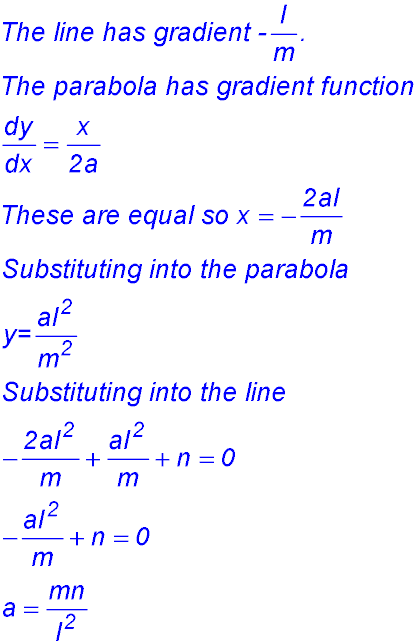Dr. J's Maths.com
Where the techniques of Maths
are explained in simple terms.

 Identification of tangents etc. 1. 2. 3.4. (i) Show that for all values of m, the line y = mx - 3m2 touches the parabola x2 = 12y. (ii) Find the values of m for which this line passes through the point (5,2). (iii) Hence determine the equations of the two tangents to the given parabola from the point (5,2). 5.Consider the line y = 6x - k and the parabola 6. More advanced. 7.Show that the constants k and l have different signs if the quadratic equationhas no real roots (). 16. Increasing fn 8. Show that the quadratic equation (a2 - b2)x2 + 2b(a - c)x + (b2 - c2) = 0 has real and rational roots for all values of x if a, b and c are rational.